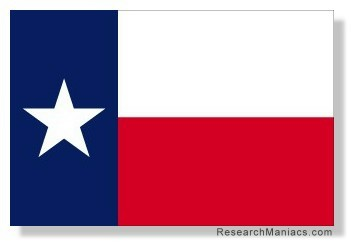How big is Texas? Question: How big is Texas? When you ask,"How big is Texas?" we assume that you are asking about the land area of Texas in square miles. Texas is 261,231.71 square miles. To put things in perspective, the entire United States is 3,531,844.39 square miles. This means that 7.40 percent of America is Texas.Research Maniacs sorted all the states by size (largest to smallest) and found Texas to be the 2nd largest state. States by size Find out how big other states are here. What is the highest point in Texas? We found the highest point in Texas.Do you know what it is? What is the lowest elevation in Texas? How low can you travel in Texas?Go here to find out! Page Summary: On this page we answered three questions: How big (large) is Texas in square miles? What percentage of America is Texas? How big is Texas compared to other states?